RS Aggarwal Class 8 Solutions Chapter 10 - Profit And Loss

RS Aggarwal Class 8 Chapter 10 - Profit And Loss Solutions Free PDF

Profit and Loss is one of the basic and most topics of mathematics. Questions from profit and loss are also asked competitive exams like UPSC, SSC and CAT. In class 8 students will learn how to calculate the profit percentage, loss percentage, cost price and selling price. Learn the important concepts of profit and loss by solving questions from RS Aggarwal for class 8.

To help students we have also provided the RS Aggarwal class 8 solutions chapter 10 profit and loss.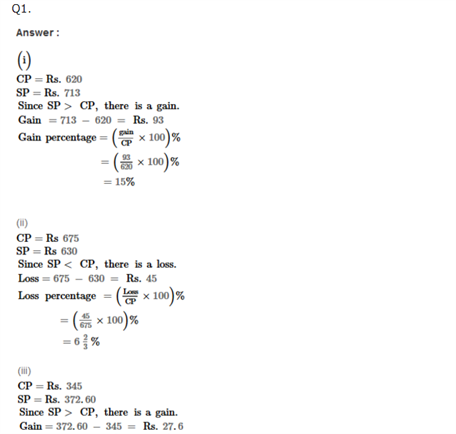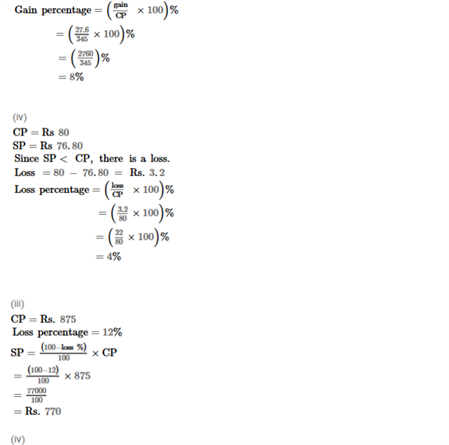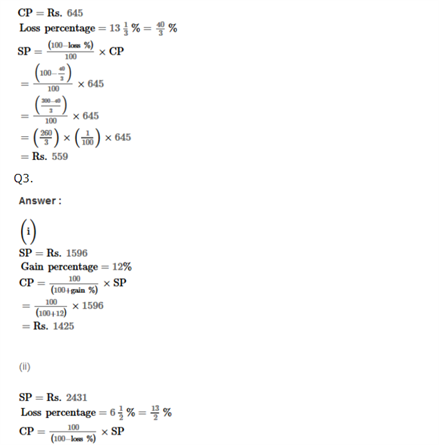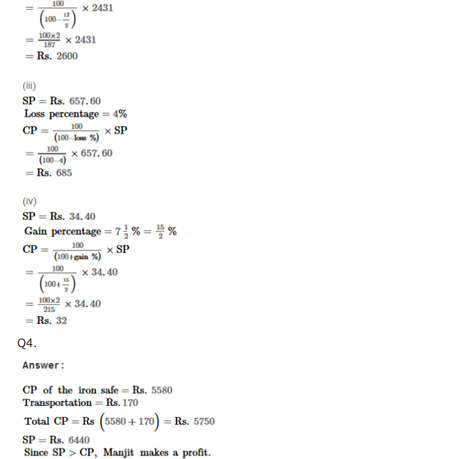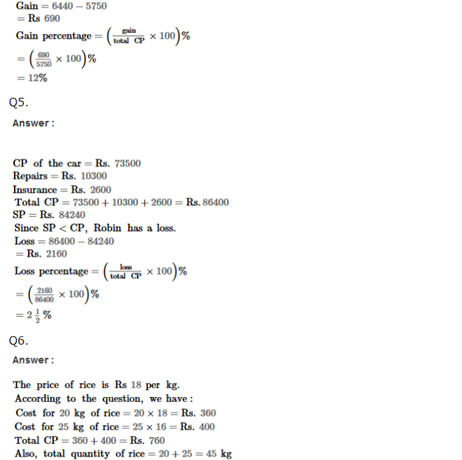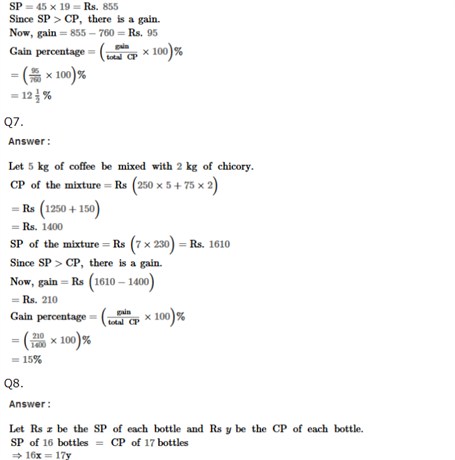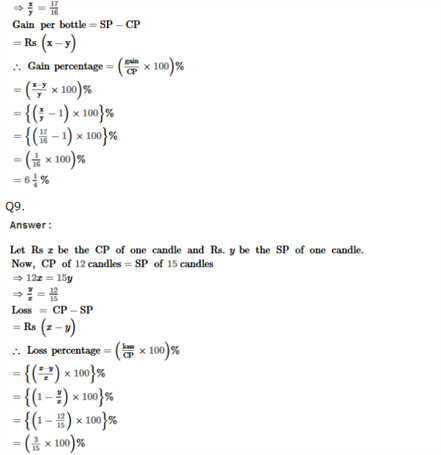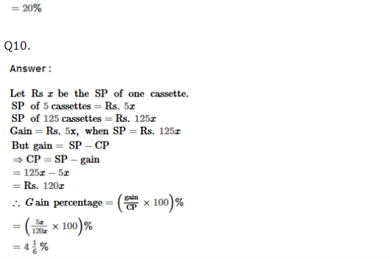Practise This Question

Water pollution is: